GET THE APP

A Spatial-Nonparametric Approach for Prediction of Claim Frequency in Motor Car Insurance

# Journal of Applied & Computational Mathematics

ISSN: 2168-9679

Open Access
Submit Manuscript arrow_forward arrow_forwardResearch Article - (2021) Volume 10, Issue 9

# A Spatial-Nonparametric Approach for Prediction of Claim Frequency in Motor Car Insurance

Kipngetich Gideon*
*Correspondence: Kipngetich Gideon, Department of Pure and Applied Sciences, Pan African University, Nairobi, Kenya, Tel: 0708366735, Email:
Department of Pure and Applied Sciences, Pan African University, Nairobi, Kenya

Received: 01-Sep-2021 Published: 24-Sep-2021 , DOI: 10.37421/2168-9679.2021.10.484
Citation: Kipngetich Gideon. "A Spatial-Nonparametric Approach for Prediction of Claim Frequency in Motor Car Insurance." J Appl J Appl Computat Math, Volume 10:9, 2021 Computat Math 9 (2021): 484.
Copyright: © 2021 Kipngetich Gideon. This is an open-access article distributed under the terms of the Creative Commons Attribution License, which permits unrestricted use, distribution, and reproduction in any medium, provided the original author and source are credited.

## Abstract

Spatial modeling has largely been applied in epidemiology and disease modeling. Different methods such as generalized linear models (GLMs), Poisson regression models, and Bayesian Models have been made available to predict the claim frequency for forthcoming years. However, due to the heterogeneous nature of policies, these methods do not produce precise and reliable prediction of future claim frequencies; these traditional statistical methods rely heavily on limiting assumptions including linearity, normality, predictor variable independence, and an established functional structure connecting the criterion and predictive variables. This study investigated how to construct a spatial nonparametric regression model estimator tor for prediction of claim frequency of insurance claims data. The study adopted a nonparametric function based on smoothing Spline in constructing the model. The asymptotic properties of the estimators; normality and consistency were derived and the inferences on the smooth function were derived. The simulation study showed that the estimator that incorporated spatial effects in predicting claims frequency is more efficient than the traditional Simultaneous Autoregressive model and Nonparametric model with Simultaneous Autoregressive error. The model estimator was applied to claims data from Cooperative Insurance Company insurance in Kenya with n = 6500 observations and the findings showed that the proposed model estimator is more efficient compared to the Local Linear fitted method, which does not account for spatial correlation. Therefore, the proposed method (Nonparametric spatial estimator) based on the findings has significant statistical improvement of the existing methods that are used for the prediction of claims. The study had a number of limitations, where the data used in the study is Lattice data (without a coordinate system); therefore, there was difficulty in classifying the claims to a specific area in the region (County).

#### Keywords

Nonparametric • SAR • Smoothing Spline • Claims • CIC • Spatial

Abbreviations

CIC: Cooperative Insurance Company

DP: Dirichlet Process

GB2: Generalized Beta of the Second Kind

PLS: Penalized Least Square

SAR: Spatial Autoregressive

LL: Local Linear

#### Introduction

Insurance has a fundamental role in providing financial protection and offering a transfer risk in exchange for an insurance premium. Therefore, estimation of the right premium for the policyholders is the noblest task in the insurance business. Insurance companies provide insurance to policyholders, and in turn, the policyholders have to pay insurance premiums through the agreed time (periodically). Due to competition in the market, charging a fair premium according to the policyholder’s expected loss is profitable for the insurer. The company will attract more customers and boost customer relationships with the insurer. The amount of premiums paid by the policyholders is determined from estimates of their expected claim frequencies and the claims’ severity. Setting precise and reliable estimates of claims frequencies has extreme importance.

Recent studies on spatial modeling have been rapidly applied in many fields; epidemiology, public health, and the Insurance sector. Different models commonly employed to fit current claims data and predict claim frequencies are Poisson regression models, generalized linear models, Credibility models, Bayesian Models. However, from the available literature, these models appear to be relatively inflexible. Essentially all models are wrong, but some are useful, which is true when the process being modeled is either not well understood, or the necessary data are not available ; the same problem of choosing the model is experienced in the modeling of the claims, Although the generalized linear models provide accurate and fast analysis of insurance data, they fall short because they are defined based on the assumptions, and an incorrect model assumption can cause model misspecification leading to erroneous results. Nonparametric models are deemed to minimize the shortcoming of these standard parametric models since fewer assumptions are made for the model, therefore, suitable for modeling insurance data which are nonlinear, nonparametric models perform better than generalized linear models (GLMs) , the only observable problems with modeling using Nonparametric models are the interpretation of some of their curves. When modeling claims and risks, we need to determine their behavior and spatial dependence, and spatial heterogeneity of the data so that the insurer can determine which areas are associated with a higher riskier when determining premiums amount to be paid.

 propose a Bayesian nonparametric approach for prediction of claims, here they found out that the model performs better compared to nonparametric GLMs in that it can capture the nonlinear random effects present in the data,  also propose a flexible nonparametric loss model for prediction of the claims, they found out that having flexible multivariate model may allow actuaries to estimate the dependence between different risk classes and different lines of business and this topic need to be explored further, and introduce the idea of using nonparametric data mining approach to modeling of the claims and prediction of risk, here the approach classify risk and predict claim size based on data. This study’s research idea was to build based on the study by [5,6] where they introduce a nonparametric spatial regression model for prediction. This study’s primary objective is to construct a spatial nonparametric estimator for the prediction of insurance claims. Therefore, this study’s main contribution was to investigate the estimator’s performance in the situation of additional covariates in the model and incorporate the aspect of spatial dependence in constructing a nonparametric estimator for the prediction of insurance claim frequencies.

The main difference between this research and [6, 7] are as follows: (1) estimate a nonparametric spatial model where estimation of the unknown trend g (·) is based on smoothing spline (2) Rather than assuming that spatial correlation takes a particular form (as in SAR), spatial correlation and spatial heterogeneity are considered simultaneously with second order stationarity (3) the estimators’ asymptotic properties under mild conditions.

#### Methods and Materials

In this section, we propose methods of estimating a claim frequency model for prediction. The basic claim frequency model is introduced, stressing the need to introduce a new method of estimating a more flexible claim model where the basic model restriction is relaxed. The spatial effects are incorporated to complete the proposed model.

Claim Model

Claim model is used a generalized linear model in modelling aggregate claims in non-life insurance . This aggregate claim model can be improved by adding a more attractive feature on the way the fit is. Defined the following terms

1. Claim severity is the total claims divided by the number of claims (average size per claim)

2. Claim frequency is the number of claims divided by the duration (average number of claims per unit time)

Most traditional claim models have assumed that the claim follows a Poisson process with a rate λ; on the other hand, claim severities follow a continuous model, and gamma distribution has been extensively used. The aggregate claim model is given by

St= X1 + X+…+ XYi

Where St is the aggregate claim amount of a given trading yearn t, Yi is random number that denotes the number of claims in a year t, Xi with i = 1,2,…Yi is the amount of ith claim realized in the year t. Some of the important assumptions as defined in the model equation (1) include

Yi ~ Poisson λ for some0 < ƛ < ∞ are iid ∀i

Yi and Xi are independent ∀i

Xi are iid ∀i Traditionally in insurance practice, the claim frequency has been modelled following Poisson distribution and Xi has the form of the loss distribution, i.e., separate gamma distribution for the claim severity . Modeling the frequency of claims, let Yi denotes the number of claims involving i policies at time t, then the total expected number of claims Y is expressed as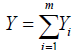m is the total number of observed policies, Yi is mostly assumed to follow discrete distribution of which Poisson distribution has been commonly used in many models. Yi depends on covariates such as the region where the policy was taken, age, sex, type of vehicle, number of claims per policy, years of policy ownership, insured cases number for a user and average claim size, then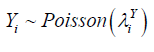Where mean is given by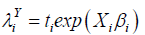Xi is covariate vector for ith observation and is assumed to have linear relationship with Yi, ti denotes the exposure time of policyholder i and βi denotes a vector of unknown regression parameters.

The assumptions on Xi and Yi are mispecifications, and if the data appear to be nonlinear, it will create a substantial modeling bias. Therefore, a nonparametric method is proposed; the main aim of this nonparametric technique is to relax highly restrictive regression function .

#### Model Estimation

The study proposes a nonparametric regression model to predict the number of claims Yi, i = 1,….n observed in region J in order to relax restrictive assumption on the distribution of number of claims and Xi covariates vector for the ith claim. Since claims in each region, J has nonlinear relation with the covariates Xi' s . The nonparametric form of the model is given by the general form [11, 12].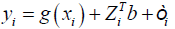G (.) is unknown nonparametric function used to model fixed effects, ZiTb

and εi for random unobserved effects. Since the form of  ZiTb = Ri   for Ri  is

Let  I = (1,...,1)Tand  n = (n1,..., nN  )Tbe two dimensional vectors. We make assumption about the spatial model as(2)

Where, i =(i1,… ,iN) in Ʌn will be referred to as site, Ri cater for the spatial effects (Random effects) and the cardinality of Ʌn is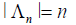.

Modeling spatial data as a finite realization of a vector stochastic process indexed by R = (R ,…,R ) R = (Ri … Rn)T follow joint Gaussian distribution here E(Ri) = 0, σ2 = var(Ri) and ∀i ∈Λn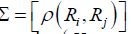correlation coefficient matrix that need to be estimated. For the vector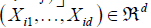Yi∈ R and

g (·), the unknown function g (·), need to be estimated for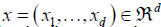, the response variable Yi is claim frequency and Xi is six dimensional vector consisting of the following explanatory variables: gender, claim amount, age of the policyholder, gender, vehicle age, model of the vehicle and age category of the policyholder.

Estimating g(x) at some point x∈Rd for Xi in the neighborhood of x, g(Xi) can be estimated by using smoothing spline . The estimator ( ) ^ g (⋅)of g (⋅) is the minimizer of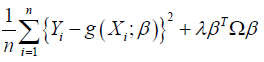(3)

Which can be represented as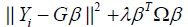where G∈Rn×n is basis matrix defined as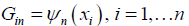Where 1, n ψ …ψ are the truncated power basis functions with knots at x1,… xn which is evaluated at the data values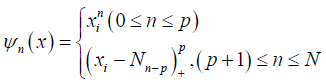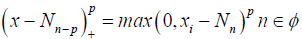where p is compact interval. p is the degree of the spline and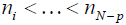are fixed points or knots in φ .

Ω∈Rn×n is the penalty matrix defined as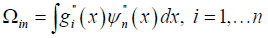Given the optimal coefficients ^β minimizing (3), the smoothing spline estimate at x is therefore defined as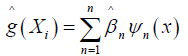The term affects shrinking components of estimation ^β towards zero. The parameter ƛ ≥ 0 is the smoothing parameter.

Each computed coefficient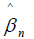corresponds to a particular basis function ψn. the term βTΩ β in (3) imparts more shrinkage on the coefficientsthat correspond to wigglier functions ψn(x) . Hence, as we increase ƛ, we are shrinking away the wiggler basis functions. Similar, to least squares regression, the coefficients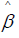minimizing (3) is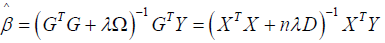The smoothing spline can be seen as linear smoother, therefore we represent as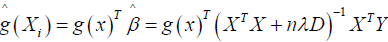(5)

which is linear combination of the points Yi, i = 1, …n and X is a design matrix with entries xi for i = 1, …,n, Y, Y is a vector of the response variables, D is a diagonal matrix with p +1 zeros on the diagonal followed by N ones and nλD is a penalty term ƛ is estimated as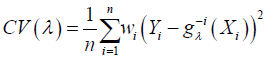(6)

Where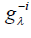is the smoothing spline estimator fitted from the data less the ith data Since Σ in model equation (2) is unknown, we assume that Ri, i = 1,2,…, n is 2nd˗ order stationary and isotopic process. Then let C(h) and γ (h) be covariogram and variogram respectively, where the distance between two locations is given by h [15,13] Then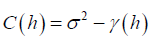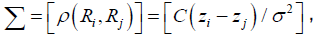while zi and zj are the spatial locations associated with error values Ri and Rj, so it is appropriate to estimate γ (h) for Σ [16,17]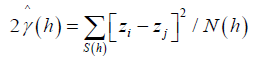(7)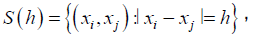N (h) is a number of distinct pairs in S (h). The characteristics of the estimator (7) can be estimated using Integrated square , given by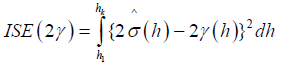Where h1 and hk are the lags . Therefore, since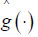has been estimated then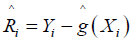Since R(zi), the error at location zi, is unobserved, this quantity must be estimated as well. We use the iterative procedure :

Obtain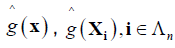by means of (4). Then put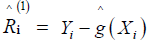Obtain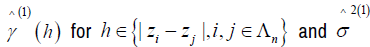Using (5), obtain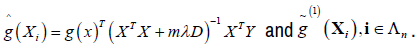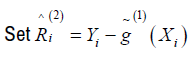and go to 2. Step 3 then produces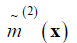and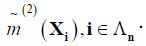Repeat this process to obtain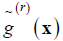and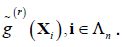The study selects ϵ > 0, i.e., ϵ = 0.001 and the procedure ends when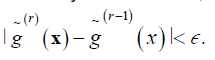As proposed by [5, 6] R2 is used to assess the performance of predictors, given by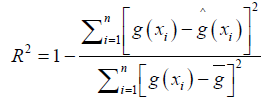Where g̅ is the mean of g(xi), i=1,…,n.

Theoretical properties of Estimator

To obtain asymptotic results, we impose the following assumptions on model (2)

A1. The random field {Xi,i ϵ Ʌn} is strictly stationary.

A2. The function g (·) is twice differentiable and its matrix of second derivatives at x denoted by g"(∙) is continuous at all x ϵ.

A3. The process Ri, i = 1,2,…, n is 2nd order stationary and isotopic, further ∃ a ϵ > 0 such that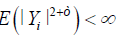for i = 1,2, … , n

Theorem 1: Asymptotic Normality

In addition to A1-A3, suppose that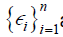are iid with mean 0 and variance σ2In then, for any xi ϵ and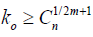for some constant C > 0 then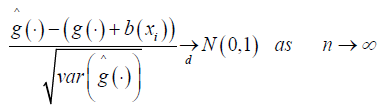(8)

Where b (xi) is asymptotic bias , given by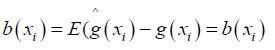Proof of Theorem 1: For m > 1, S (m,t) is a set of spline functions with knots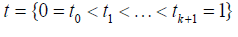of step functions with jumps at the knots and for m ≥ 2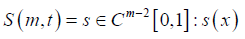Expressing function in S (m,t) in terms of B-splines for fixed m and t, let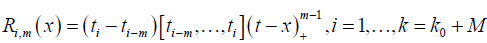Where [ti-m, …, ti]f denote the mth – order divide difference of the function f and ti = tmin(max(i,0),ko +1) for any i = 1- M, …, k we assume that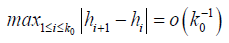And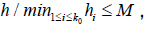where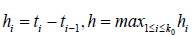and M > 0 (predetermined constant) this assumption ensure that M-1 < k0h < M , which is necessary for numerical assumptions

Let Dn (x) be an empirical distribution function of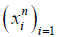with a positive continuous density d(x) this implies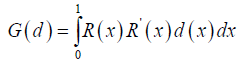Then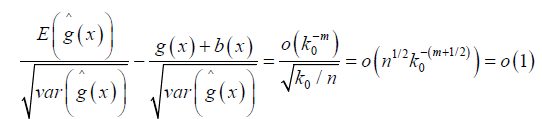Thus equation (8) follows if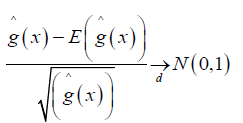we have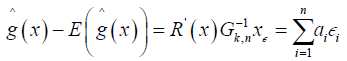Where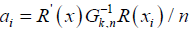the required Lindeberg-Feller conditions, it suffices to verify that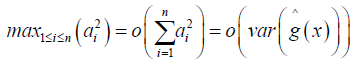we also have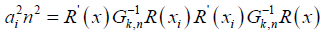Finally, equation (8) follows from the assumption that k0 / n → ∞ hence the prove.

Theorem 2

Consistency: From theorem 1, we can establish the asymptotic consistencywhere for ϵ > 0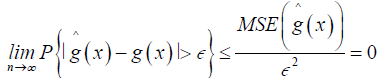(9)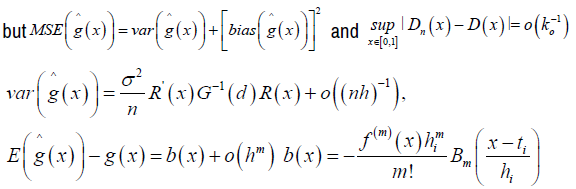Where Bo (x) =1,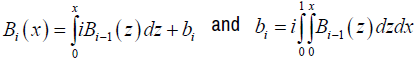is the ith Bernoulli number . From equation (9)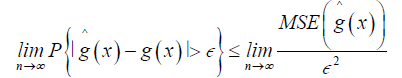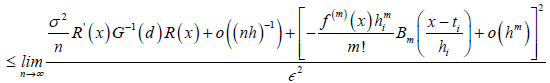(10)

As n→ ∞ the numerator terms in RHS of equation (10) collapse to zero therefore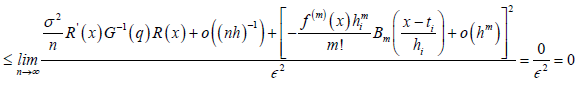Hence the prove of equation (9), therefore we conclude that the estimator is consistent to the true function g(x) of equation model (2).

Data Description

The study used data from Cooperative Insurance Company’s motor thirdparty liability insurance for 2018 and 2019. 6500 policies are included in the data. The following policy information was used: the area where the policy was purchased, policyholder age, gender, vehicle type, number of claims, years of policy ownership, claim amount, and insured cases number. Age is categorized into three categorize; Old (50 > years), young (<25 years) and Middle age (25-50) (Table 1).

Table 1 shows that there is a very large number of observations has no claims in claims dataset where the maximum number of claims made in a region were 4 in an observation. The histogram in (Figure 1) of the observed claims shows that the distribution of claims is skewed to the right, therefore there is element of over-dispersion in the data due to large number of zeros.

Table 1. Claim frequency table for the data.

No. of Claims Frequency of Observations Percentage of Observations
0 4015 61.8
1 1967 30.3
2 431 6.6
3 71 1.1
4 16 0.2

#### Simulation Results

This section describes the simulation process and the results of the method proposed in this research. This study proposed a model proposed by [6,13] given by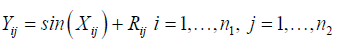Where Yij are the observations and Xij is a saptial process which represents the explanatory variables and Rij is the term for spatial effects? The semivariogram is estimated as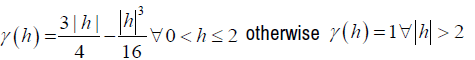Where h is the distance between 2 locations zi and zj in 2-dimensional space Xij is a spatial process and follows a mean 0 and second order stationary gaussian process, for this reason we use spectral method to simulate Xij given by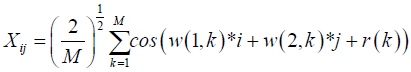w(i, k ) k =1,…,M are iid normally distributed and are independent of r(k) iid uniform random variables on [―π, π] as n → ∞, Xij converges to a gaussian ergodic process . The matrix of random errors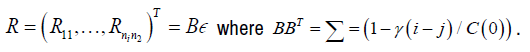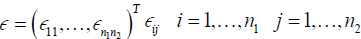are iid normally distributed.

We set the basis function as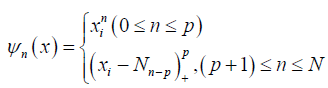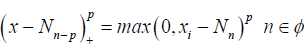where Φ is compact interval. p is the degree of the spline and ni < …<nN-p are fixed knots in Φ To measure performance of estimators, we use R2 given by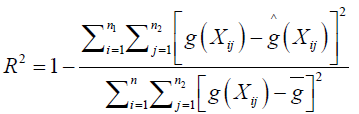where g̅ is the sample mean of g(Xi), i = 1, .., n. The closer R2 to 1 the better the performance of the estimators. Comparing the performance of the proposed method with the other immediate existing methods such as nonparametric spatial models with general correlation structures a method proposed by  where estimation was based on kernel estimation and the nonparametric spatial autoregressive (SAR) method under which R = ρ WR + ϵ proposed by .

Table 2 shows the simulation results of R2 values from 100 simulations. We can see that R2 for the proposed method is the largest and closer to 1; this demonstrates the superior performance of the proposed method.

Table 2. R2 for model 1 over 100 simulations under different sample sizes.

Data  Sample sizes
Method  10 × 10 20 × 20
SAR 0.7483 0.9587
NonPar(Wang 2017) 0.7585 0.9691
Proposed Method 0.8217 0.9962

Analysis for claims data

The study considers the claims data from CIC insurance observed in different parts of 42 counties of Kenya to illustrate the performance of the proposed method procedures, which exclude the 5 counties from the northern part of the country since the information regarding the 5 counties was not available. Since we are mainly interested in predicting the claims frequency, the study considers a set of 6500 observations data observed from 42 counties. Let Yi denote the claim frequency, and Xi = (X1, …., X6) T be a vector which consists of the following explanatory variables: gender, claim amount, age of the policyholder, gender, vehicle age, model of the vehicle and age category of the policyholder. By using the following model where, we can predict claim frequencies.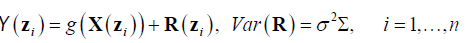Where Y(zi):i = 1, … , n is the observations (claims) in region zi associated with independent variable X(zi) in region zi, R(zi) is the unobserved error in region zi and g(.) is the estimated function. The observations are from a random process observed over a countable collection of spatial regions (county). The data at a particular location typically represent the entire region. Claims data resides on an irregular lattice, with each site representing an entire county . Using R2 to measure the performance of the predictor, R2 is defined as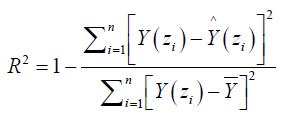From the analysis of the data, the results were presented in the following table (3).

From table 3 the R2 of LL (local linear method) without unobserved random correlation errors is 0.7125 the proposed method outperforms LL model with R2 value of 0.9484. In presence of spatial effects, as the iterations increase the performance of the proposed method is significantly better than that of the LL. It is observed that R2 increases very little in columns 5 and 6.

Table 3. R2 larger towards 1 the better) for claims data.

Method LL Proposed Method 1st  iteration 2nd iteration 3rd iteration 4th iteration
R2 0.7125 0.9484 0.9531 0.9543 0.9544 0.9544

#### Discussion

The construction of the estimator aims at obtaining a robust method for the prediction of claims frequencies. The proposed method adopts a nonparametric approach where smooth function estimated using smoothing spline accounts for the fixed effects.

A random effect (spatial error) was added to the model to improve the prediction. From the simulation study, two methods; Nonparametric model with SAR error  and Nonparametric model with the correlated error, which uses kernel in the estimation  were compared to the proposed method. The results showed that the proposed method has a significantly better prediction performance of 0.9962 (99.62 efficiencies) compared to the two methods, which have R2 values 0.9587(95.87% efficiency) and 0.9691(96.91% efficiency), respectively. The proposed method was applied to the Cooperative Insurance Company (CIC) claims data-set. The findings showed that the proposed model is more efficient with R2 of 0.9544, which is closer to 1 (100% efficiency) in predicting the claims frequencies compared to the Local Linear fitted model with no account for spatial effects with R2 of 0.7125(71.25% efficiency). The proposed method could now be applied to other classes of insurance claims due to its efficiency and capability to capture the nonlinear effects and spatial effects characterized by claims data.

#### Conclusion

The idea of deriving an appropriate estimator in predicting frequency claims in the insurance industry has gained more interest in finance and statistical research. Many researchers heavily rely on parametric estimators; however, the insurance datasets have some aspect of non-linearity. Hence, researchers in statistics are developing nonparametric estimators with spatial error to improve the prediction based on existing parametric models. The study constructed a spatial nonparametric estimator for predicting claim frequencies in motor insurance, and the theoretical properties of the estimators were derived. The study established the theoretical properties of the proposed estimators under mild conditions. The study found that the proposed nonparametric spatial method is more efficient from the simulation results and application of the method to claims data with 6500 observations than the Local Linear fitting models and nonparametric model with Spatial Autoregressive error in the prediction of claims frequencies. Therefore, based on the results, the proposed method can be applied in predicting claims frequencies due to its efficiency and capability to capture the nonlinear effects characterized by claims data.

Limitations & Recommendations for Further Studies

Some additional exogenous variables may influence claim frequency. For example, the environmental factors among other institutional factors. It is important to investigate how each of the factors affects the output variable. Thus, a more robust spatial estimator for studying the relationship between an output variable and input variables may be constructed in subsequent studies using the proposed method. The data set used in the study involves policies for cars taken by the CIC insurance company. However, in the insurance, there are also policies for life insurance. Hence, further studies should consider the use of other claims data sets (i.e., for life and property claims data sets) in demonstrating the applications of the constructed estimator and also consider developing a more efficient nonparametric package to map Lattice observations under this proposed method.

Limitations of the Study

The data used in the study is Lattice data (without a coordinate system); therefore, there was difficulty in classifying the claims to a specific area in the region (County).

Acknowledgments

I would like to thank the Pan African University for their support and enabling environment also the CIC company for accepting to share their claims datasets which aid in accomplishing the objective of the study.

Conflicts of Interest

The authors declare no conflict of interest.

Appendix

The CIC insurance company did not provide URL link for accessing the data instead they provide an excel sheet containing the data.

#### Journal Highlights

###### Citations: 1282

Journal of Applied & Computational Mathematics received 1282 citations as per Google Scholar report